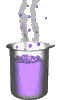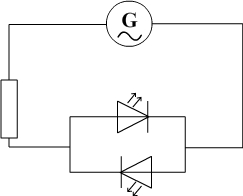Science class online
Chemistry and physics lessons

# Electricity

Free online electricity lessons for elementary school,  middle school and high school.Science class Chemistry Electricity Optics Mechanics Electricity lessons Electrical components -Two-terminal electronic components - Basic electrical components - Diodes - What's a resistor ? - How to determine a resistor value ? - Resistors effects in circuits - How to use a resistor ? - Characteristic curve of a resistor How to build simple circuits and draw diagrams - How to build a basic electrical circuit - How to draw diagrams of electric circuits The electric current - Conductors and insulators - Direction of electric current in a circuit - The dangers of electricity - Current intensity - units how to measure current ? The Voltage - The voltage and its units - How to measure a voltage ? - Voltage in open and closed circuits - Rated current and voltage for a lamp Alternating voltage and current - Alternating currents effects on led - What are alternating current and voltage ? - Periodic alternating voltage and its properties - Oscilloscope - Oscillogram - Frequency - Measuring RMS voltage with a voltmeter Serie circuits - What's a serie circuit ? - Series circuit properties - Short circuit in  series circuit - Current law in series - circuits - Voltage law in series circuits Parallel circuits - What's a parallel circuit ? - Some parallel circuits properties - Short circuits in parallel circuits - Nodes and branches in parallel circuits - Current laws in parallel circuits - Voltage laws in parallel circuits Laws of electricity - Ohm's law - Current laws in series circuits - Voltage laws in series circuits - Current laws in parallel circuits - Voltage laws in parallel circuits Generating electricity - Voltage for coil wires What is an alternator ? - Alternating currents and voltages - Generating electricity in power plants Electric power and energy - Electric power and power rating - Electric power consumption by an electrical device - Relationship between - Electric power and energy

 _____________________________________________________   _____________________________________________________

## Alternative currents and voltages

### Alternating currents effects on LEDs

 The change in direction of an alternating current can be observed in a circuit with a alternative generator, two LEDs connected in parallel and opposite directions and a resistor to protect the LED. Circuit DiagramReminders: - A bypass LED lets current pass and shines. - A blocking LED doesn't let current pass and doesn't shine. We observe in this circuit that one of the diodes shine while the other remains off. Regularly the situation is reversed: the LED that shone goes off while the other LED lights up. The two LEDs are connected in opposite directions and when one is in the direction from the other is blocking. When the current direction is reversed the blocking LED allows current to flow and the one that was in the forward direction becomes blocking. Comment: - When changes of direction are too fast, the blinkings are no longer perceptible to the human eye and LEDs seem to shine continuously. - The small wave below the symbol of the generator is the symbol for an alternative quantity. ______________________________________ ______________________________________
____________________________________

____________________________________